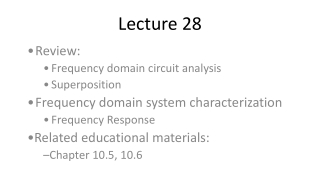DownloadDownload PresentationLecture 28

# Lecture 28

Download Presentation## Lecture 28

- - - - - - - - - - - - - - - - - - - - - - - - - - - E N D - - - - - - - - - - - - - - - - - - - - - - - - - - -
##### Presentation Transcript

1. Lecture 28 Review: Frequency domain circuit analysis Superposition Frequency domain system characterization Frequency Response Related educational materials: Chapter 10.5, 10.6

2. Note during summary that we will be changing our mindset, rather than doing anything fundamentally new • I will keep re-introducing the same example circuit, but with minor modifications and extensions

3. Frequency domain analysis – review • The analysis techniques we used for time domain analysis of resistive networks are applicable to frequency domain analysis of general circuits • E.g. KVL, KCL, circuit reduction, nodal analysis, mesh analysis, Thevenin’s and Norton’s Theorems… • In general, we simply need to: • Subsitute impedances for resistances • Use phasor voltages & currents in place of time domain voltages & currents

4. Superposition • Superposition in frequency domain: • If multiple signals exist at different frequencies, superposition is the only valid frequency domain approach • Effects of individual sources can be analyzed independently in the frequency domain • Summation of individual contributions must be done in the time domain (unless all contributions have same frequency)

5. Example 1 – Superposition • Determine the steady-state voltage across the inductor in the circuit below

6. In previous slide, show time domain superposition. V(t) = v1(t) +v2(t) • Note that we can determine v1(t) and v2(t) individually using frequency domain analysis • We can’t however, superimpose these individually, for reasons that will be made clear on the next slide

7. Example 1 – continued • The associated frequency domain circuits are shown below:

8. Derive the phasors V1 and V2 on the previous slide • Point out that they can’t be added directly – they don’t even apply to the same frequency domain circuit • We will superimpose the results in the time domain

9. Example 1 – continued again • Superimpose results in the time domain:

10. On previous slide, V1 has frequency of 9 rad/sec and V2 has frequency of 3 rad/sec

11. Notes on superposition • Superposition of sinusoidal signals is extremely important in circuit analysis! • In later courses, we will see that (nearly any) signal can be represented as a superposition of sinusoidal signals, using Fourier Series and Fourier Transforms • If we determine the circuit’s response to each of these sinusoids and superimpose them, we can determine the circuit’s response to (nearly any) input function • We will spend the next couple of lectures looking at circuit responses to multiple sinusoidal inputs

12. Example 2 • Determine the capacitor voltage, v(t), for the circuit below if vS1(t) = 3cos(2t+20) and vS2(t) = 5cos(2t-45)

13. On the previous chart, draw single-source circuit and point out that VS = vs1+vs2 and v(t)=v1+v2 • Draw two frequency-domain circuits ; note that they both have the same appearance

14. Try a trick to do example 2 easily • Let’s look at an “arbitrary” input with the same frequency as our individual inputs:

15. Determine input-output relationship (gain, phase) => point out that this is only good for 2 rad/sec frequency sinusoids

16. Example 2 – continued • Now we can use our trick to easily determine the circuit’s response to both inputs (since they are at the same frequency)

17. Important result • The steady-state sinusoidal response of a circuit, at a frequency 0, can be characterized by two values: • Gain: output-to-input amplitude ratio • Phase difference: difference between the output and input phases

18. On previous slide, annotate output to show on block diagram: • Y = H*U, where H is a complex number that depends only on frequency • Circuit “looks like” H, at the frequency of interest

19. Example 3 – Multiple input frequencies • Determine the capacitor voltage, v(t), for the circuit below if vS1(t) = 3cos(2t+30) and vS2(t) = 5cos(4t+60)

20. Annotate slide to show two frequency-domain circuits; note that they have different frequency-domain characteristics • I don’t want to analyze two circuits, so let’s leave frequency in the circuit model!

21. Try a similar trick with example 3 • Let’s characterize the circuit’s gain and phase as a function of frequency:

22. Example 3 – continued • Now we can use our trick to determine the circuit’s response to both inputs and superimpose the results vS1(t) = 3cos(2t+30) vS2(t) = 5cos(4t+60)

23. On the previous slide, write H, U, and Y as functions of frequency: • H(j2), U(j2), Y(j2) • H(j4), U(j4), Y(j4) • Note that we analyzed the circuit only once!

24. Super-important result • The steady-state sinusoidal response of a circuit is characterized by the frequency response, H(j) • Magnitude response: output-to-input amplitude ratio vs. frequency • Phase response: difference between the output and input phases as a function of frequency

25. Frequency Response • In the time domain, we characterize systems by the differential equation relating the input and output • In the frequency domain, we characterize systems by their frequency response • Magnitude response and phase response give the gain and phase difference relating the input and output • The frequency of the signal (rather than time) is now our independent variable